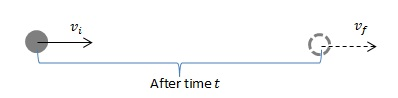# Difference Between Kinematics and Dynamics

Kinematics and dynamics are branches of mechanics, which is the study of forces and motion in physics.

## Kinematics

Kinematics is the study of motion of particles and bodies, without taking into account the factors that cause motion. Kinematics takes into account quantities such as displacement, velocity, acceleration.

For further reading: What is Kinematics in Physics

## Dynamics

Dynamics is the study of motion, along with the factors that cause motion. Calculations in dynamics, therefore, involve masses and forces. Consequently, study of quantities such as momenta can be considered as a part of dynamics.

Linear momentum$\vec{p}$ of a body is defined as the product of the body’s mass and velocity:$\vec{p} = m\vec{v}$

An external force$\vec{F}$ acting on a body causes the body to accelerate. The force is equal to the rate of change of momentum of the body. This is, in fact, Newton’s Second Law of Motion:$\vec{F} = \frac{\mathrm{d} \vec{p}}{\mathrm{d} t}$

If the mass of the body remains constant, then$\vec{F} = m\frac{\mathrm{d} \vec{v}}{\mathrm{d} t}$ and,$\vec{F} = m\vec{a}$

## Difference Between Kinematics and Dynamics: An exampleFor simplicity, let us consider a particle that is being accelerated along a straight line, with a constant acceleration$a$. The particle has an initial velocity of$v_{i}$ and after a time$t$, the velocity of the particle is$v_{f}$. Using equations of motion for a particle with constant acceleration, we can calculate the final velocity to be:$v_{f} = v_{i} + at$

This is a simple use of kinematics: we haven’t dealt with the causes of motion, we have simply made a calculation with regards to kinematic quantities.

In dynamics, we would also consider the causes of motion. For instance, for the above particle, we would like to know its mass, and how much of a force was needed to cause the particle’s velocity to change.For instance, if the particle had a mass$m$, then according to Newton’s second law, a resultant force$F = ma=m\frac{v_f-v_i}{t}$

would have been required to cause the required velocity change.

In addition, dynamics also takes into account the rotation of bodies. Therefore, study of quantities such as torques, moments of inertia and angular momenta can also be classified as dynamics.